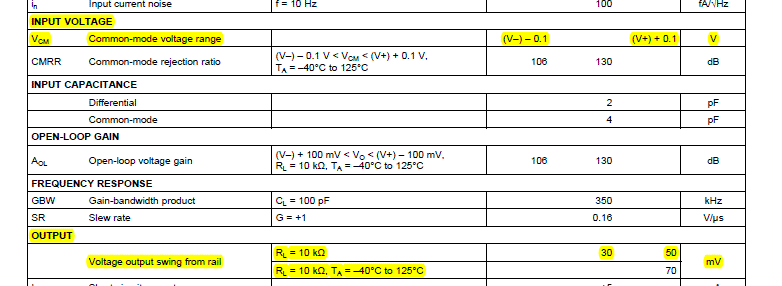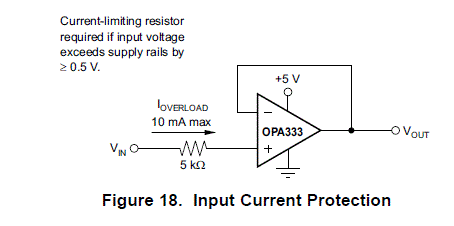If you have a related question, please click the "Ask a related question" button in the top right corner. The newly created question will be automatically linked to this question.

• TI Thinks Resolved

# OPA2333: What is the output voltage when the input voltage is less than the output minimum voltage?

Part Number: OPA2333

The OPA2333 input voltage and output voltage are as shown in the manual. What is the output voltage when the input voltage is less than the minimum output voltage?

At this point, the op amp indicator is down, or the output voltage is 30mV.

For example, when the input is 0V, 10mV, 20mV, what is the output voltage?

The test data of the single-supply buffer circuit is as follows: input 0mV, output is 0mV; input 5mV, output is 5mV; input 10mV, output is 10mV; input 20mV, output is 20mV.

I tested four pieces and the same conclusion. Because the test uses a common multimeter, the error is too large and the specific accuracy is not calculated.

I think that when the input is below the minimum output voltage, the op amp indicator will drop, not the output minimum output voltage.• Hello Rengui,

The OPA333 output voltage swing is the limiting factor when the input common-mode voltage (VCM) is set at the extremes of its range. The OPA333 output voltage is limited to about 50 mV (typical) from the rails as the Electrical Characteristics table you included show. Thus, if you set the VCM to 0, 10 mV, or 20 mV the output can't move any closer to the supply rails than typically 50 mV. If the output is unloaded, or very lightly loaded, it may swing very close to 0 V before saturation occurs.

If the output is driven to its maximum swing of ~50 mV from V+ or GND, it is no longer operating in its linear region but instead in saturation. The output will remain in saturation until VCM is changed to a level that corresponds to the output's linear operating region.

One CMOS operational amplifier that can swing to about 1 mV of the supply rails (100 k load) is the OPA340. However, its electrical specifications are considerably different from the OPA333.

Regards, Thomas

Precision Amplifiers Applications Engineering

• Rengui,

A violation of the input common-mode voltage range will cause variety of problems including offset, bandwidth, noise, IB change beyond what is specified in the datasheet and therefore the output will also change depending on the close-loop configuration - please study the material in the TI Precision Labs course under following link:Marek Lis, MGTS
Sr Application Engineer
Precision Analog - TI Tucson

• In reply to Marek Lis:

Rengui,

We haven't heard back from you so we assume this answered your question. If not, just post another reply below.

Thanks
Dennis